$$\newcommand{\id}{\mathrm{id}}$$ $$\newcommand{\Span}{\mathrm{span}}$$ $$\newcommand{\kernel}{\mathrm{null}\,}$$ $$\newcommand{\range}{\mathrm{range}\,}$$ $$\newcommand{\RealPart}{\mathrm{Re}}$$ $$\newcommand{\ImaginaryPart}{\mathrm{Im}}$$ $$\newcommand{\Argument}{\mathrm{Arg}}$$ $$\newcommand{\norm}{\| #1 \|}$$ $$\newcommand{\inner}{\langle #1, #2 \rangle}$$ $$\newcommand{\Span}{\mathrm{span}}$$

# 1.10: Functions (Review)

•• Contributed by Jay Abramson
• Principal Lecturer (School of Mathematical and Statistical Sciences) at Arizona State University
• Publisher: OpenStax CNX
$$\newcommand{\vecs}{\overset { \rightharpoonup} {\mathbf{#1}} }$$ $$\newcommand{\vecd}{\overset{-\!-\!\rightharpoonup}{\vphantom{a}\smash {#1}}}$$$$\newcommand{\id}{\mathrm{id}}$$ $$\newcommand{\Span}{\mathrm{span}}$$ $$\newcommand{\kernel}{\mathrm{null}\,}$$ $$\newcommand{\range}{\mathrm{range}\,}$$ $$\newcommand{\RealPart}{\mathrm{Re}}$$ $$\newcommand{\ImaginaryPart}{\mathrm{Im}}$$ $$\newcommand{\Argument}{\mathrm{Arg}}$$ $$\newcommand{\norm}{\| #1 \|}$$ $$\newcommand{\inner}{\langle #1, #2 \rangle}$$ $$\newcommand{\Span}{\mathrm{span}}$$ $$\newcommand{\id}{\mathrm{id}}$$ $$\newcommand{\Span}{\mathrm{span}}$$ $$\newcommand{\kernel}{\mathrm{null}\,}$$ $$\newcommand{\range}{\mathrm{range}\,}$$ $$\newcommand{\RealPart}{\mathrm{Re}}$$ $$\newcommand{\ImaginaryPart}{\mathrm{Im}}$$ $$\newcommand{\Argument}{\mathrm{Arg}}$$ $$\newcommand{\norm}{\| #1 \|}$$ $$\newcommand{\inner}{\langle #1, #2 \rangle}$$ $$\newcommand{\Span}{\mathrm{span}}$$

## 1.1: Functions and Function Notation

For the exercises 1-4, determine whether the relation is a function.

1) $$\{(a,b),(c,d),(e,d)\}$$

function

2) $$\{(5,2),(6,1),(6,2),(4,8)\}$$

3) $$y^2+4=x$$$,$for $$x$$ the independent variable and $$y$$ the dependent variable

not a function

4) Is the graph in the Figure below a function?For the exercises 5-6, evaluate the function at the indicated values: $$f(-3); f(2); f(-a); -f(a); f(a+h)$$

5) $$f(x)=-2x^2+3x$$

$$f(-3)=-27; f(2)=-2;f(-a)=-2a^2-3a;-f(a)=2a^2-3a;f(a+h)=-2a^2+3a-4ah+3h-2h^2$$

6) $$f(x)=2|3x-1|$$

For the exercises 7-8, determine whether the functions are one-to-one.

7) $$f(x)=-3 x+5$$

one-to-one

8) $$f(x)=|x-3|$$

For the exercises 9-11, use the vertical line test to determine if the relation whose graph is provided is a function.

9)function

10)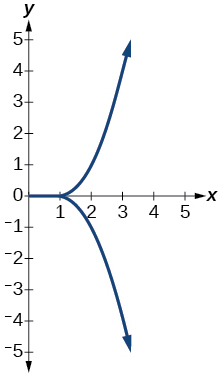11)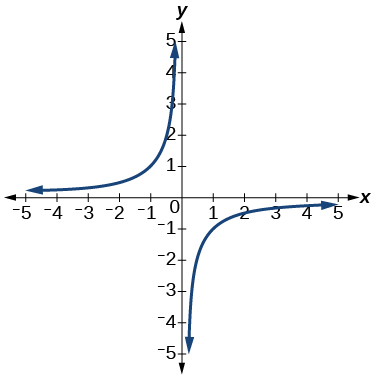function

For the exercises 12-13, graph the functions.

12) $$f(x)=|x+1|$$

13) $$f(x)=x^{2}-2$$For the exercises 14-17, use the Figure below to approximate the values.14) $$f(2)$$

15) $$f(-2)$$

$$2$$

16) If $$f(x)=-2$$, then solve for $$x$$

17) If $$f(x)=1$$, then solve for $$x$$

$$x=-1.8$$ or $$x=1.8$$

For the exercises 18-19, use the function $$h(t)=-16 t^{2}+80t$$ to find the values.

18) $$\dfrac{h(2)-h(1)}{2-1}$$

19) $$\dfrac{h(a)-h(1)}{a-1}$$

$$\dfrac{-64+80 a-16 a^{2}}{-1+a}=-16 a+64$$

## 1.2: Domain and Range

For the exercises 1-4, find the domain of each function, expressing answers using interval notation.

1) $$f(x)=\dfrac{2}{3 x+2}$$

2) $$f(x)=\frac{x-3}{x^{2}-4 x-12}$$

$$(-\infty,-2) \cup(-2,6) \cup(6, \infty)$$

3)

4) Graph this piecewise function: $$f(x)=\left\{\begin{array}{ll}{x+1} & {x<-2} \\ {-2 x-3} & {x \geq-2}\end{array}\right.$$## 1.3: Rates of Change and Behavior of Graphs

For the exercises 1-3, find the average rate of change of the functions from $$x=1$$ to $$x=2$$

1) $$f(x)=4 x-3$$

2) $$f(x)=10 x^{2}+x$$

$$31$$

3) $$f(x)=-\dfrac{2}{x^{2}}$$

For the exercises 4-6, use the graphs to determine the intervals on which the functions are increasing, decreasing, or constant.

4)increasing $$(2, \infty)$$; decreasing $$(-\infty, 2)$$

5)6)increasing $$(-3,1)$$; constant $$(-\infty,-3) \cup(1, \infty)$$

7) Find the local minimum of the function graphed in Exercise 4.

8) Find the local extrema for the function graphed in Exercise 5.

local minimum $$(-2,-3)$$; local maximum $$(1,3)$$

9) For the graph in the Figure in Exercise 10, the domain of the function is $$[-3,3]$$. The range is $$[-10,10]$$. Find the absolute minimum of the function on this interval.

10) Find the absolute maximum of the function graphed in the Figure below.$$(-1.8,10)$$

## 1.4: Composition of Functions

For the exercises 1-5, find $$(f \circ g)(x)$$ and $$(g \circ f)(x)$$ for each pair of functions.

1) $$f(x)=4-x, g(x)=-4x$$

2) $$f(x)=3 x+2, g(x)=5-6x$$

$$(f \circ g)(x)=17-18 x ;(g \circ f)(x)=-7-18x$$

3) $$f(x)=x^{2}+2 x, g(x)=5 x+1$$

4) $$f(x)=\sqrt{x+2}, g(x)=\dfrac{1}{x}$$

$$(f \circ g)(x)=\sqrt{\dfrac{1}{x}+2} ;(g \circ f)(x)=\dfrac{1}{\sqrt{x+2}}$$

5) $$f(x)=\dfrac{x+3}{2}, g(x)=\sqrt{1-x}$$

For the exercises 6-9, find $$(f \circ g)$$ and the domain for $$(f \circ g)(x)$$ for each pair of functions.

6) $$f(x)=\frac{x+1}{x+4}, g(x)=\frac{1}{x}$$

$$(f \circ g)(x)=\dfrac{1+x}{1+4 x}, x \neq 0, x \neq-\dfrac{1}{4}$$

7) $$f(x)=\dfrac{1}{x+3}, g(x)=\dfrac{1}{x-9}$$

8) $$f(x)=\dfrac{1}{x}, g(x)=\sqrt{x}$$

$$(f \circ g)(x)=\frac{1}{\sqrt{x}}, x>0$$

9) $$f(x)=\frac{1}{x^{2}-1}, g(x)=\sqrt{x+1}$$

For the exercises 10-11, express each function $$H$$ as a composition of two functions $$f$$ and $$g$$ where $$H(x)=(f \circ g)(x)$$

10) $$H(x)=\sqrt{\frac{2 x-1}{3 x+4}}$$

sample: $$g(x)=\dfrac{2 x-1}{3 x+4}; f(x)=\sqrt{x}$$

11) $$H(x)=\dfrac{1}{\left(3 x^{2}-4\right)^{-3}}$$

## 1.5: Transformation of Functions

For the exercises 1-8, sketch a graph of the given function.

1) $$f(x)=(x-3)^{2}$$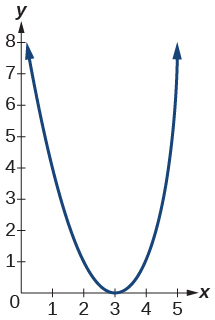2) $$f(x)=(x+4)^{3}$$

3) $$f(x)=\sqrt{x}+5$$4) $$f(x)=-x^{3}$$

5) $$f(x)=\sqrt{-x}$$6) $$f(x)=5 \sqrt{-x}-4$$

7) $$f(x)=4[|x-2|-6]$$8) $$f(x)=-(x+2)^{2}-1$$

For the exercises 9-10, sketch the graph of the function $$g$$ if the graph of the function $$f$$ is shown in the Figure below.9) $$g(x)=f(x-1)$$10) $$g(x)=3 f(x)$$

For the exercises 11-12, write the equation for the standard function represented by each of the graphs below.

11)$$f(x)=|x-3|$$

12)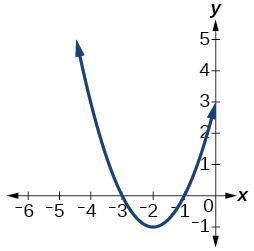For the exercises 13-15, determine whether each function below is even, odd, or neither.

13) $$f(x)=3 x^{4}$$

even

14) $$g(x)=\sqrt{x}$$

15) $$h(x)=\frac{1}{x}+3 x$$

odd

For the exercises 16-18, analyze the graph and determine whether the graphed function is even, odd, or neither.

16)17)even

18)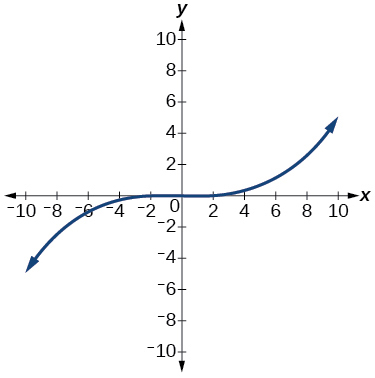## 1.6: Absolute Value Functions

For the exercises 1-3, write an equation for the transformation of $$f(x)=|x|$$.

1)$$f(x)=\dfrac{1}{2}|x+2|+1$$

2)3)$$f(x)=-3|x-3|+3$$

For the exercises 4-6, graph the absolute value function.

4) $$f(x)=|x-5|$$

5) $$f(x)=-|x-3|$$6) $$f(x)=|2 x-4|$$

For the exercises 7-8, solve the absolute value equation.

7) $$|x+4|=18$$

$$x=-22, x=14$$

8) $$\left|\dfrac{1}{3} x+5\right|=\left|\dfrac{3}{4} x-2\right|$$

For the exercises 9-10, solve the inequality and express the solution using interval notation.

9) $$|3 x-2|<7$$

$$\left(-\dfrac{5}{3}, 3\right)$$

10) $$\left|\dfrac{1}{3} x-2\right| \leq 7$$

## 1.7: Inverse Functions

For the exercises 1-2, find $$f^{-1}(x)$$ for each function.

1) $$f(x)=9+10 x$$

2) $$f(x)=\dfrac{x}{x+2}$$

$$f^{-1}(x)=\dfrac{-2 x}{x-1}$$

3) For the following exercise, find a domain on which the function $$f$$ is one-to-one and non-decreasing. Write the domain in interval notation. Then find the inverse of $$f$$ restricted to that domain. $f(x)=x^{2}+1$

4) Given $$f(x)=x^{3}-5$$ and $$g(x)=\sqrt{x+5}$$ :

1. Find $$f(g(x))$$ and $$g(f(x))$$.
2. What does the answer tell us about the relationship between $$f(x)$$ and $$g(x) ?$$
1. $$f(g(x))=x$$ and $$g(f(x))=x$$
2. This tells us that $$f$$ and $$g$$ are inverse functions

For the exercises 5-8, use a graphing utility to determine whether each function is one-to-one.

5) $$f(x)=\dfrac{1}{x}$$

The function is one-to-one.6) $$f(x)=-3 x^{2}+x$$

The function is not one-to-one.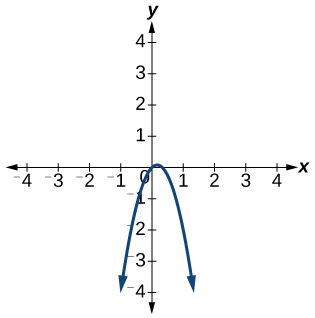7) If $$f(5)=2,$$ find $$f^{-1}(2)$$

$$5$$

8) If $$f(1)=4,$$ find $$f^{-1}(4)$$

## Practice Test

For the exercises 1-2, determine whether each of the following relations is a function.

1) $$y=2 x+8$$

The relation is a function.

2) $$\{(2,1),(3,2),(-1,1),(0,-2)\}$$

For the exercises 3-4, evaluate the function $$f(x)=-3 x^{2}+2 x$$ at the given input.

3) $$f(-2)$$

$$-16$$

4) $$f(a)$$

5) Show that the function $$f(x)=-2(x-1)^{2}+3$$ is not one-to-one.

The graph is a parabola and the graph fails the horizontal line test.

6) Write the domain of the function $$f(x)=\sqrt{3-x}$$ in interval notation.

7) Given $$f(x)=2 x^{2}-5 x,$$ find $$f(a+1)-f(1)$$

$$2 a^{2}-a$$

8) Graph the function $$f(x)=\left\{\begin{array}{ccc}{x+1} & {\text { if }} & {-2<x<3} \\ {-x} & {\text { if }} & {x \geq 3}\end{array}\right.$$

9) Find the average rate of change of the function $$f(x)=3-2 x^{2}+x$$ by finding $$\dfrac{f(b)-f(a)}{b-a}$$

$$-2(a+b)+1$$

For the exercises 10-11, use the functions $$f(x)=3-2 x^{2}+x$$ and $$g(x)=\sqrt{x}$$ to find the composite functions.

10) $$(g \circ f)(x)$$

11) $$(g \circ f)(1)$$

$$\sqrt{2}$$

12) Express $$H(x)=\sqrt{5 x^{2}-3 x}$$ a composition of two functions, $$f$$ and $$g,$$ where $$(f \circ g)(x)=H(x)$$

For the exercises 13-14, graph the functions by translating, stretching, and/or compressing a toolkit function.

13) $$f(x)=\sqrt{x+6}-1$$14) $$f(x)=\dfrac{1}{x+2}-1$$

For the exercises 15-17, determine whether the functions are even, odd, or neither.

15) $$f(x)=-\dfrac{5}{x^{2}}+9 x^{6}$$

even

16) $$f(x)=-\dfrac{5}{x^{3}}+9 x^{5}$$

17) $$f(x)=\dfrac{1}{x}$$

odd

18) Graph the absolute value function $$f(x)=-2|x-1|+3$$.

19) Solve $$|2 x-3|=17$$.

$$x=-7$$ and $$x=10$$

20) Solve $$-\left|\dfrac{1}{3} x-3\right| \geq 17$$ . Express the solution in interval notation.

For the exercises 21-22, find the inverse of the function.

21) $$f(x)=3 x-5$$

$$f^{-1}(x)=\dfrac{x+5}{3}$$

22) $$f(x)=\dfrac{4}{x+7}$$

For the exercises 23-26, use the graph of $$g$$ shown in the Figure below.

23) On what intervals is the function increasing?

$$(-\infty,-1.1)$$ and $$(1.1, \infty)$$

24) On what intervals is the function decreasing?

25) Approximate the local minimum of the function. Express the answer as an ordered pair.

$$(1.1,-0.9)$$

26) Approximate the local maximum of the function. Express the answer as an ordered pair.

For the exercises 27-29, use the graph of the piecewise function shown in the Figure below.

27) Find $$f(2)$$.

$$f(2)=2$$

28) Find $$f(-2)$$.

29) Write an equation for the piecewise function.

$$f(x)=\left\{\begin{array}{cl}{|x|} & {\text { if } x \leq 2} \\ {3} & {\text { if } x>2}\end{array}\right.$$

For the exercises 30-35, use the values listed in the Table below.

 $$x$$ $$F(x)$$ 0 1 1 3 2 5 3 7 4 9 5 11 6 13 7 15 8 17

30) Find $$F(6)$$.

31) Solve the equation $$F(x)=5$$

$$x=2$$

32) Is the graph increasing or decreasing on its domain?

33) Is the function represented by the graph one-to-one?

34) Find $$F^{-1}(15)$$.
35) Given $$f(x)=-2 x+11,$$ find $$f^{-1}(x)$$.
$$f^{-1}(x)=-\dfrac{x-11}{2}$$